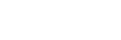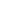Explore all Jira open source software, libraries, packages, source code, cloud functions and APIs.

## Popular New Releases in Jira

 jira v1.0.27 Release jira v3.2.0 go-jira v1.15.1 atlassian-python-api Adjust Confluence Cloud and Jira JQL methods jira-cli v0.3.0
 jirav1.0.27 Release jirav3.2.0 go-jirav1.15.1 atlassian-python-apiAdjust Confluence Cloud and Jira JQL methods jira-cliv0.3.0

1

13 Libraries

1065

2

7 Libraries

577

3

7 Libraries

552

4

7 Libraries

5763

5

7 Libraries

166

6

5 Libraries

19

7

5 Libraries

263

8

5 Libraries

1211

9

4 Libraries

24

10

4 Libraries

95

1

13 Libraries

1065

2

7 Libraries

577

3

7 Libraries

552

4

7 Libraries

5763

5

7 Libraries

166

6

5 Libraries

19

7

5 Libraries

263

8

5 Libraries

1211

9

4 Libraries

24

10

4 Libraries

95

## Trending Kits in Jira

No Trending Kits are available at this moment for Jira

## Trending Discussions on Jira

QUESTION

Regex validation for time tracking

I am trying to validate a string the way it is done in Jira in Javascript. I'm trying to replicate how it is validated in Jira. I am guessing I could do this with Regex but I am not sure how.

A user can type a string in the format of "1d 6h 30m" which would mean 1 day, 6 hours, 30 minutes. I do not need the weeks for my use case. I want to show an error if the user uses an invalid character (anything except 'd','h','m', or ' '). Also the string must separate the time durations by spaces and ideally I would like to force the user to enter the time durations in descending order meaning '6h 1d' would be invalid because the days should come first. Also the user does not have to enter all information so '30m' would be valid.

This is my code for getting the days, hours and minutes which seems to work. I just need help with the validation part.

``````1let time = '12h 21d 30m'; //example
2let times = time.split(' ');
3let days = 0;
4let hours = 0;
5let min = 0;
6for(let i = 0; i &lt; times.length; i++) {
7    if (times[i].includes('d')){
8        days = times[i].split('d');
9    }
10    if (times[i].includes('h')){
11        hours = times[i].split('h');
12    }
13    if (times[i].includes('m')){
14        min = times[i].split('m');
15    }
16}
17console.log(days);
18console.log(hours);
19console.log(min);
20``````

Based on your comment, I have added a validation regex to be run first before running the match regex.

For validation, you want``````1let time = '12h 21d 30m'; //example
2let times = time.split(' ');
3let days = 0;
4let hours = 0;
5let min = 0;
6for(let i = 0; i &lt; times.length; i++) {
7    if (times[i].includes('d')){
8        days = times[i].split('d');
9    }
10    if (times[i].includes('h')){
11        hours = times[i].split('h');
12    }
13    if (times[i].includes('m')){
14        min = times[i].split('m');
15    }
16}
17console.log(days);
18console.log(hours);
19console.log(min);
20/^(\d+[d]\s+)?(\d+[h]\s+)?(\d+[m]\s+)?(\d+[s]\s+|\$)?/
21``````

For extracting values, you want``````1let time = '12h 21d 30m'; //example
2let times = time.split(' ');
3let days = 0;
4let hours = 0;
5let min = 0;
6for(let i = 0; i &lt; times.length; i++) {
7    if (times[i].includes('d')){
8        days = times[i].split('d');
9    }
10    if (times[i].includes('h')){
11        hours = times[i].split('h');
12    }
13    if (times[i].includes('m')){
14        min = times[i].split('m');
15    }
16}
17console.log(days);
18console.log(hours);
19console.log(min);
20/^(\d+[d]\s+)?(\d+[h]\s+)?(\d+[m]\s+)?(\d+[s]\s+|\$)?/
21/([\d]+[dhms]\s+|\$)/g
22``````

You can then use String.match with this regular expression, iterating through all the matches add adding time based on the time letter at the end

Community Discussions contain sources that include Stack Exchange Network

QUESTION

Regex validation for time tracking

I am trying to validate a string the way it is done in Jira in Javascript. I'm trying to replicate how it is validated in Jira. I am guessing I could do this with Regex but I am not sure how.

A user can type a string in the format of "1d 6h 30m" which would mean 1 day, 6 hours, 30 minutes. I do not need the weeks for my use case. I want to show an error if the user uses an invalid character (anything except 'd','h','m', or ' '). Also the string must separate the time durations by spaces and ideally I would like to force the user to enter the time durations in descending order meaning '6h 1d' would be invalid because the days should come first. Also the user does not have to enter all information so '30m' would be valid.

This is my code for getting the days, hours and minutes which seems to work. I just need help with the validation part.

``````1let time = '12h 21d 30m'; //example
2let times = time.split(' ');
3let days = 0;
4let hours = 0;
5let min = 0;
6for(let i = 0; i &lt; times.length; i++) {
7    if (times[i].includes('d')){
8        days = times[i].split('d');
9    }
10    if (times[i].includes('h')){
11        hours = times[i].split('h');
12    }
13    if (times[i].includes('m')){
14        min = times[i].split('m');
15    }
16}
17console.log(days);
18console.log(hours);
19console.log(min);
20``````

Based on your comment, I have added a validation regex to be run first before running the match regex.

For validation, you want``````1let time = '12h 21d 30m'; //example
2let times = time.split(' ');
3let days = 0;
4let hours = 0;
5let min = 0;
6for(let i = 0; i &lt; times.length; i++) {
7    if (times[i].includes('d')){
8        days = times[i].split('d');
9    }
10    if (times[i].includes('h')){
11        hours = times[i].split('h');
12    }
13    if (times[i].includes('m')){
14        min = times[i].split('m');
15    }
16}
17console.log(days);
18console.log(hours);
19console.log(min);
20/^(\d+[d]\s+)?(\d+[h]\s+)?(\d+[m]\s+)?(\d+[s]\s+|\$)?/
21``````

For extracting values, you want``````1let time = '12h 21d 30m'; //example
2let times = time.split(' ');
3let days = 0;
4let hours = 0;
5let min = 0;
6for(let i = 0; i &lt; times.length; i++) {
7    if (times[i].includes('d')){
8        days = times[i].split('d');
9    }
10    if (times[i].includes('h')){
11        hours = times[i].split('h');
12    }
13    if (times[i].includes('m')){
14        min = times[i].split('m');
15    }
16}
17console.log(days);
18console.log(hours);
19console.log(min);
20/^(\d+[d]\s+)?(\d+[h]\s+)?(\d+[m]\s+)?(\d+[s]\s+|\$)?/
21/([\d]+[dhms]\s+|\$)/g
22``````

You can then use String.match with this regular expression, iterating through all the matches add adding time based on the time letter at the end

Community Discussions contain sources that include Stack Exchange Network

## Tutorials and Learning Resources in Jira

Tutorials and Learning Resources are not available at this moment for Jira